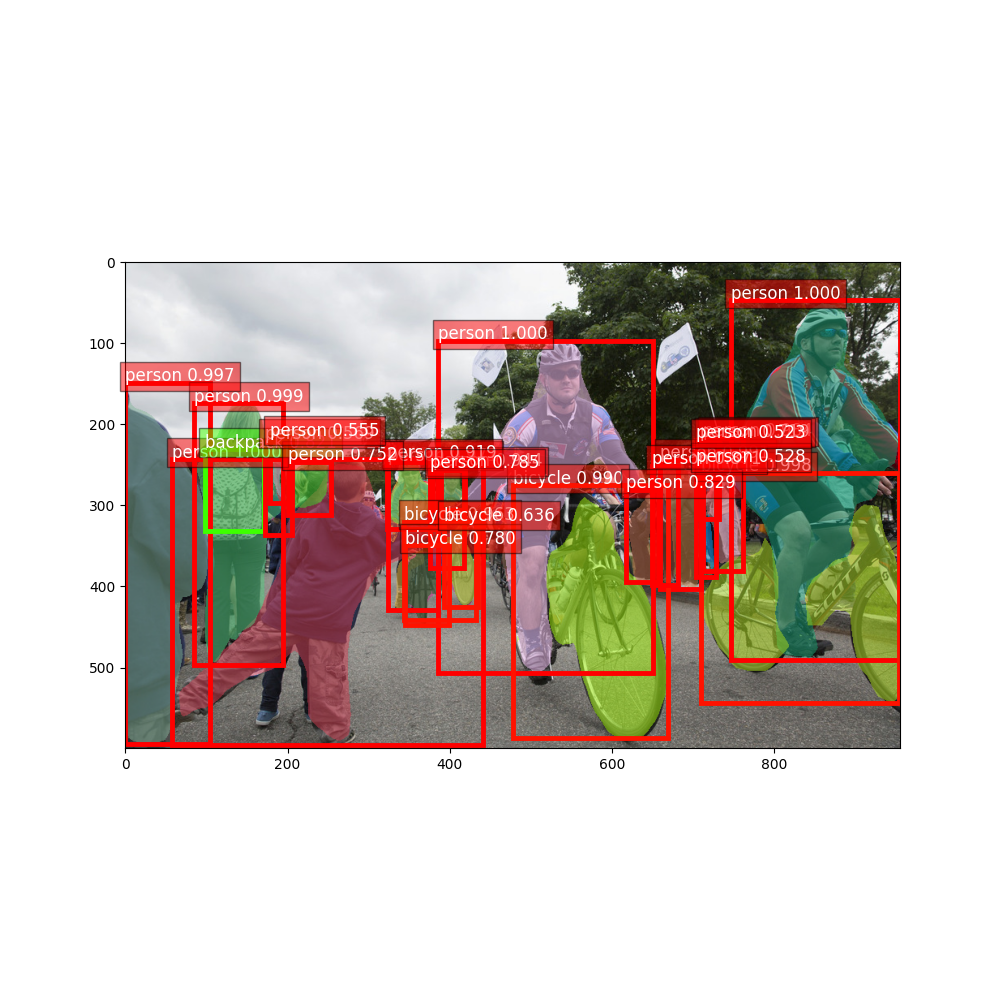# 1. Predict with pre-trained Mask RCNN models¶

Mask RCNN networks are extensions to Faster RCNN networks. `gluoncv.model_zoo.MaskRCNN` is inherited from `gluoncv.model_zoo.FasterRCNN`. It is highly recommended to read 02. Predict with pre-trained Faster RCNN models first.

First let’s import some necessary libraries:

```from matplotlib import pyplot as plt
from gluoncv import model_zoo, data, utils
```

Let’s get an Mask RCNN model trained on COCO dataset with ResNet-50 backbone. By specifying `pretrained=True`, it will automatically download the model from the model zoo if necessary. For more pretrained models, please refer to Model Zoo.

The returned model is a HybridBlock `gluoncv.model_zoo.MaskRCNN` with a default context of cpu(0).

```net = model_zoo.get_model('mask_rcnn_resnet50_v1b_coco', pretrained=True)
```

## Pre-process an image¶

The pre-processing step is identical to Faster RCNN.

Next we download an image, and pre-process with preset data transforms. The default behavior is to resize the short edge of the image to 600px. But you can feed an arbitrarily sized image.

You can provide a list of image file names, such as ```[im_fname1, im_fname2, ...]``` to `gluoncv.data.transforms.presets.rcnn.load_test()` if you want to load multiple image together.

This function returns two results. The first is a NDArray with shape (batch_size, RGB_channels, height, width). It can be fed into the model directly. The second one contains the images in numpy format to easy to be plotted. Since we only loaded a single image, the first dimension of x is 1.

Please beware that orig_img is resized to short edge 600px.

```im_fname = utils.download('https://github.com/dmlc/web-data/blob/master/' +
'gluoncv/detection/biking.jpg?raw=true',
path='biking.jpg')
```

Out:

```Downloading biking.jpg from https://github.com/dmlc/web-data/blob/master/gluoncv/detection/biking.jpg?raw=true...

0%|          | 0/244 [00:00<?, ?KB/s]
100%|##########| 244/244 [00:00<00:00, 32729.23KB/s]
```

## Inference and display¶

The Mask RCNN model returns predicted class IDs, confidence scores, bounding boxes coordinates and segmentation masks. Their shape are (batch_size, num_bboxes, 1), (batch_size, num_bboxes, 1) (batch_size, num_bboxes, 4), and (batch_size, num_bboxes, mask_size, mask_size) respectively. For the model used in this tutorial, mask_size is 14.

Object Detection results

We can use `gluoncv.utils.viz.plot_bbox()` to visualize the results. We slice the results for the first image and feed them into plot_bbox:

Plot Segmentation

`gluoncv.utils.viz.expand_mask()` will resize the segmentation mask and fill the bounding box size in the original image. `gluoncv.utils.viz.plot_mask()` will modify an image to overlay segmentation masks.

```ids, scores, bboxes, masks = [xx.asnumpy() for xx in net(x)]

# paint segmentation mask on images directly
width, height = orig_img.shape, orig_img.shape